# Basic Trigonometric Identities Pythagorean Identity Trigonometric Ratios Consider

• Slides: 21Basic Trigonometric Identities Pythagorean Identity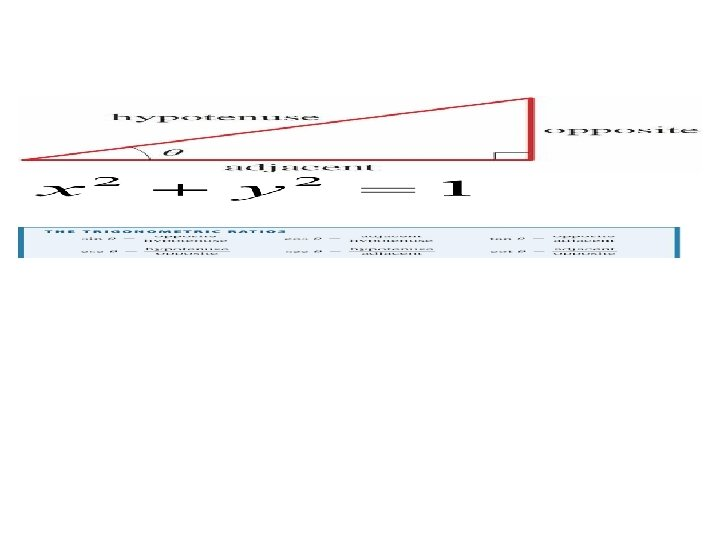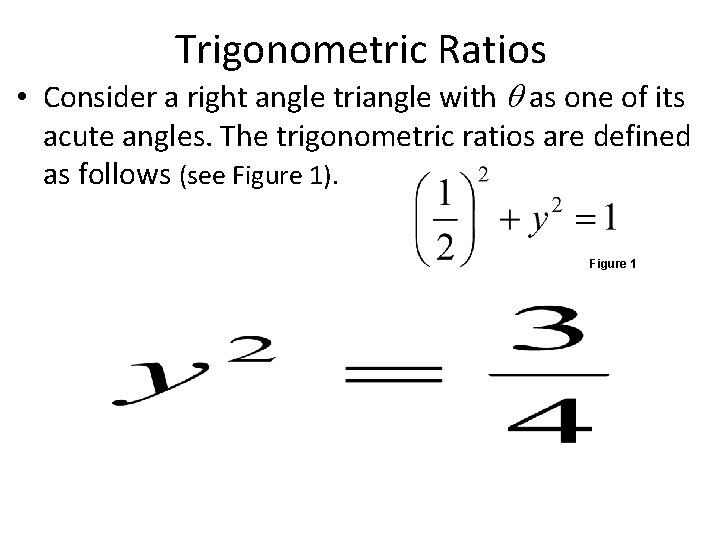Trigonometric Ratios • Consider a right angle triangle with as one of its acute angles. The trigonometric ratios are defined as follows (see Figure 1). Figure 1Unit Circle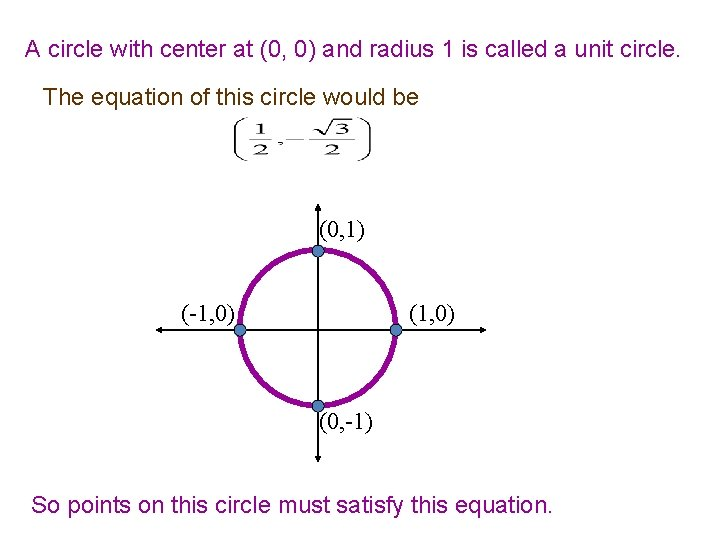A circle with center at (0, 0) and radius 1 is called a unit circle. The equation of this circle would be (0, 1) (-1, 0) (0, -1) So points on this circle must satisfy this equation.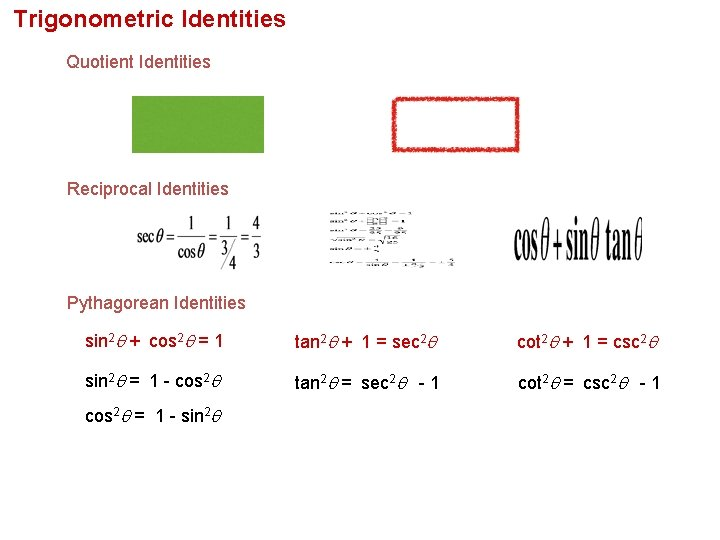Trigonometric Identities Quotient Identities Reciprocal Identities Pythagorean Identities sin 2 + cos 2 = 1 tan 2 + 1 = sec 2 cot 2 + 1 = csc 2 sin 2 = 1 - cos 2 tan 2 = sec 2 - 1 cot 2 = csc 2 - 1 cos 2 = 1 - sin 2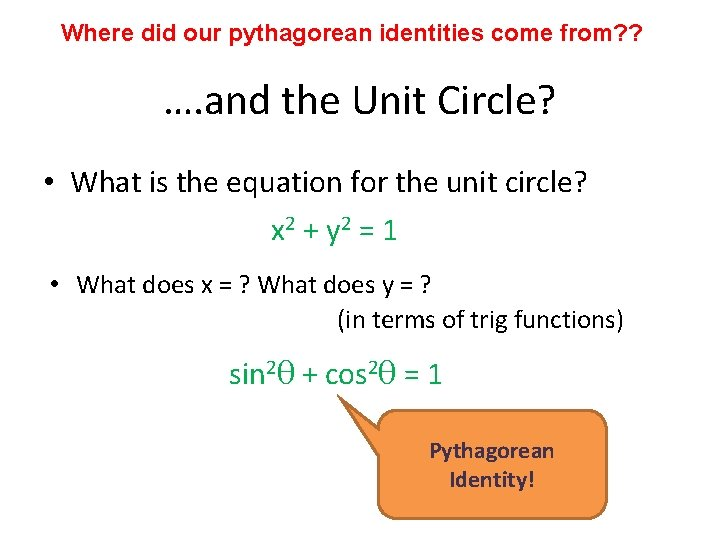Where did our pythagorean identities come from? ? …. and the Unit Circle? • What is the equation for the unit circle? x 2 + y 2 = 1 • What does x = ? What does y = ? (in terms of trig functions) sin 2θ + cos 2θ = 1 Pythagorean Identity!Take the Pythagorean Identity and discover a new one! Hint: Try dividing everything by cos 2θ sin 2θ + cos 2θ = 1. cos 2θ tan 2θ + 1 = sec 2θ Quotient Identity another Pythagorean Identity Reciprocal IdentityTake the Pythagorean Identity and discover a new one! Hint: Try dividing everything by sin 2θ + cos 2θ = 1. sin 2θ 1 + cot 2θ = csc 2θ Quotient Identity a third Pythagorean Identity Reciprocal IdentitySimplifying trig Identity Example: Simplify tanx cosx sin x tanx cos x tanxcosx = sin xSimplifying trig Identity Example: simplify sec x csc x 1 cos sec x csc 1 x sin x = 1 sinx x cos x 1 = sin x cos x = tan x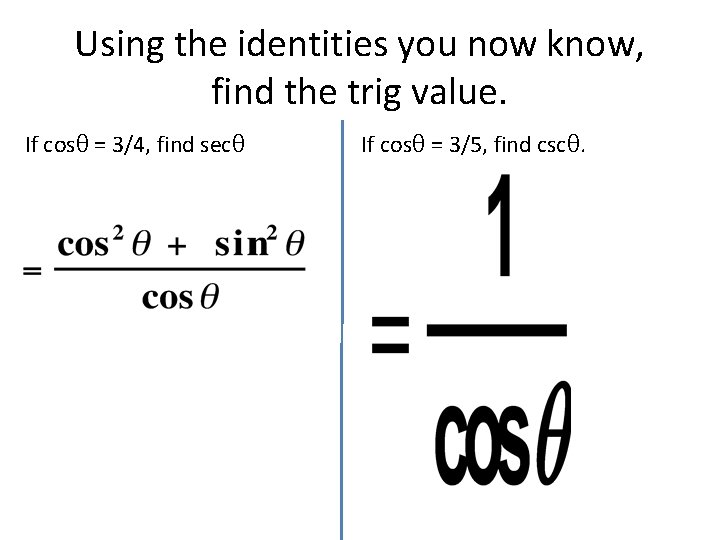Using the identities you now know, find the trig value. If cosθ = 3/4, find secθ If cosθ = 3/5, find cscθ.Simplifying Trigonometric Expressions Identities can be used to simplify trigonometric expressions. Simplify. a) b)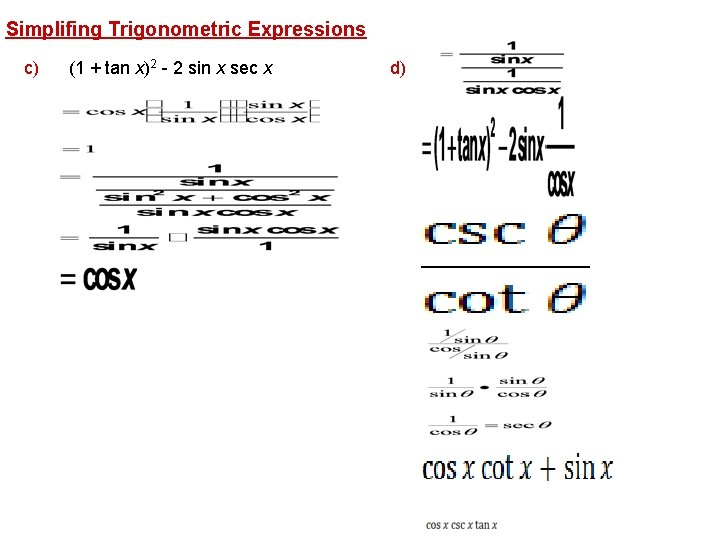Simplifing Trigonometric Expressions c) (1 + tan x)2 - 2 sin x sec x d)Simplify each expression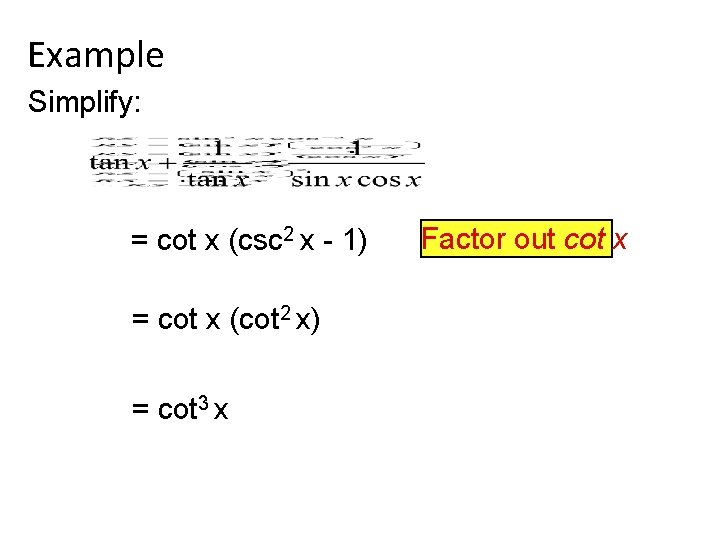Example Simplify: = cot x (csc 2 x - 1) = cot x (cot 2 x) = cot 3 x Factor out cot xExample Simplify: = sin x (sin x) + cos x 2 = sin x + (cos x)cos x = sin 2 x + cos 2 x cos x = 1 cos x = sec x Use quotient identity Simplify fraction with LCD Simplify numerator Use pythagorean iden Use reciprocal identity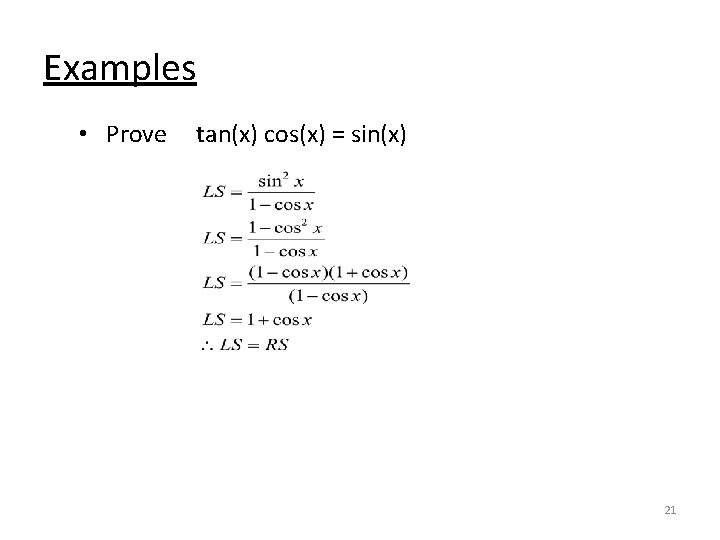Examples • Prove tan(x) cos(x) = sin(x) 21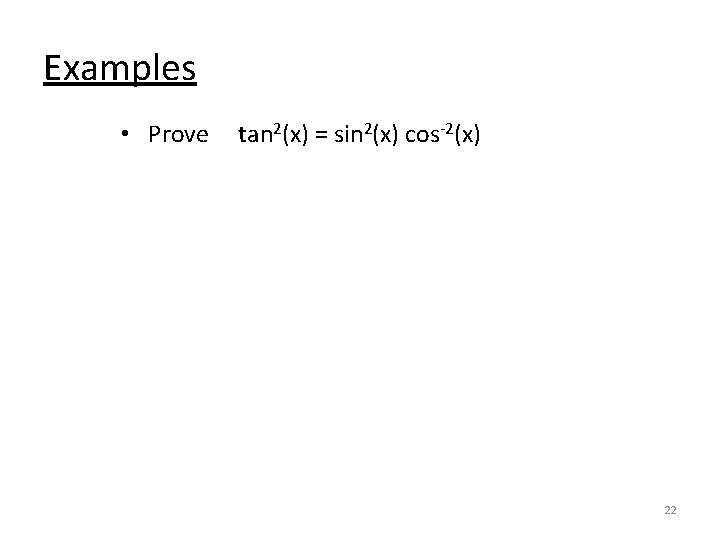Examples • Prove tan 2(x) = sin 2(x) cos-2(x) 22Examples • Prove 23Examples • Prove 24